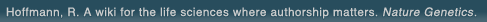# The world's first wiki where authorship really matters (Nature Genetics, 2008). Due credit and reputation for authors. Imagine a global collaborative knowledge base for original thoughts. Search thousands of articles and collaborate with scientists around the globe.

wikigene or wiki gene protein drug chemical gene disease author authorship tracking collaborative publishing evolutionary knowledge reputation system wiki2.0 global collaboration genes proteins drugs chemicals diseases compound
Hoffmann, R. A wiki for the life sciences where authorship matters. Nature Genetics (2008)# Simultaneous partitioning and hydrolysis kinetics of amoxicillin and ampicillin.

The kinetics of ampicillin and amoxicillin partitioning with simultaneous acid-catalyzed hydrolysis were studied in a stirred transfer cell containing isobutanol as the extract and aqueous hydrochloric acid (0.1-0.5 N) as the raffinate at 37 degrees. Biexponential data for the concentration in both the raffinate (C1) and the extract ( C2) as a function of time were analyzed simultaneously by nonlinear regression to estimate the apparent first-order rate constant for transfer from hydrochloric acid to isobutanol (k '12), the reverse transfer constant (k '21), and the hydrolysis rate constant (k). Agreement between k values determined in the presence of simultaneous partitioning and those determined in the absence of partitioning (k app) verified the nonlinear estimates. Apparent partition coefficients, which represent the values that would be obtained in the absence of hydrolysis K'D = C1 infinity/C2 infinity), were estimated from K'D = k'12/k'21. During terminal monoexponential loss, where C1 approximately equal to Y'e-beta t and C2 approximately equal to Z'e-beta t, the kinetically controlled C2/C1 ratio (r) is described by [K'12/K'21-beta)], which decreases with decreasing kappa values until r approaches K'D. The difference between the terminal concentration ratio, r, and its corresponding partition coefficient, K'D, is a measure of the degree to which kinetic processes control distribution. Both ampicillin and amoxicillin showed kinetic control of the distribution ratios in 0.5 N HCl, where the hydrolysis rate constant was significant relative to the distribution rate constants. Ampicillin had r approximately equal to 1.74 and K'D approximately equal to 0.92; amoxicillin had r approximately equal to 0.95 and K'D approximately equal to 0.65. As the (K'12 + K'21/k ratio increased, the r values approached K'D so that in 0.1 N HCl, r approximately K'D = 0.33 for amoxicillin and r approximately 0.6 and K'D approximately 0.56 for ampicillin. In general, amoxicillin distribution rate constants (K'12 + K'21) were roughly twice those of ampicillin, whereas ampicillin K'D and r values were nearly double those of amoxicillin. Thus, the kinetic and thermodynamic rank orders are opposite. This result may have implications in drug design via molecular modification.

## References

1. Simultaneous partitioning and hydrolysis kinetics of amoxicillin and ampicillin. Tomlinson, E., Notari, R.E., Byron, P.R. Journal of pharmaceutical sciences. (1980) [Pubmed]